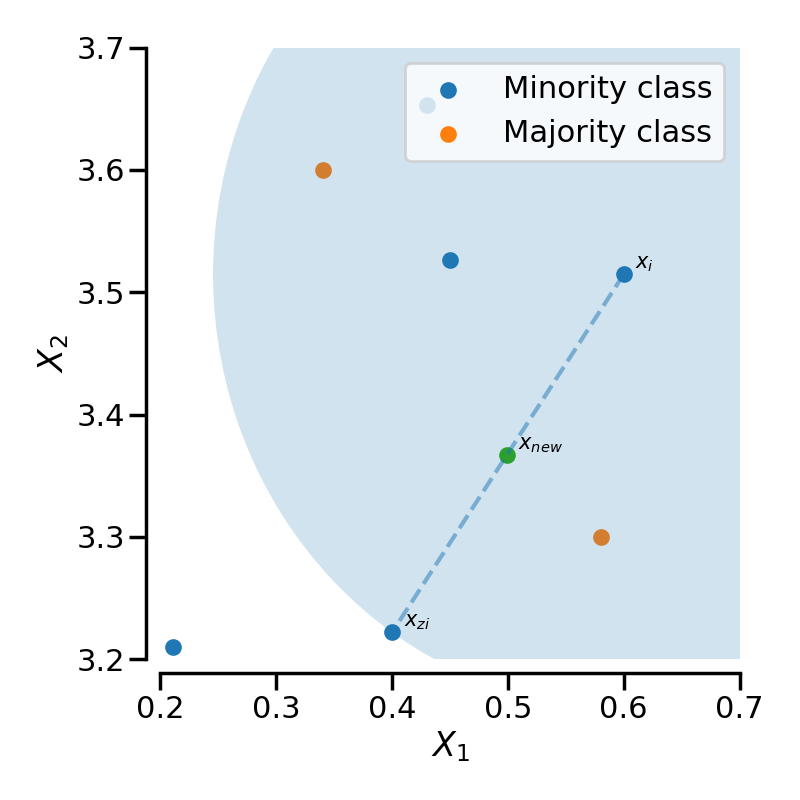# Sample generator used in SMOTE-like samplers#

This example illustrates how a new sample is generated taking into account the neighbourhood of this sample. A new sample is generated by selecting the randomly 2 samples of the same class and interpolating a point between these samples.

```# Authors: Guillaume Lemaitre <g.lemaitre58@gmail.com>
```
```print(__doc__)

import matplotlib.pyplot as plt
import numpy as np
import seaborn as sns

sns.set_context("poster")

rng = np.random.RandomState(18)

f, ax = plt.subplots(figsize=(8, 8))

# generate some data points
y = np.array([3.65284, 3.52623, 3.51468, 3.22199, 3.21])
z = np.array([0.43, 0.45, 0.6, 0.4, 0.211])
y_2 = np.array([3.3, 3.6])
z_2 = np.array([0.58, 0.34])

# plot the majority and minority samples
ax.scatter(z, y, label="Minority class", s=100)
ax.scatter(z_2, y_2, label="Majority class", s=100)

idx = rng.randint(len(y), size=2)
annotation = [r"\$x_i\$", r"\$x_{zi}\$"]

for a, i in zip(annotation, idx):
ax.annotate(a, (z[i], y[i]), xytext=tuple([z[i] + 0.01, y[i] + 0.005]), fontsize=15)

# draw the circle in which the new sample will generated
radius = np.sqrt((z[idx] - z[idx]) ** 2 + (y[idx] - y[idx]) ** 2)

# plot the line on which the sample will be generated
ax.plot(z[idx], y[idx], "--", alpha=0.5)

# create and plot the new sample
step = rng.uniform()
y_gen = y[idx] + step * (y[idx] - y[idx])
z_gen = z[idx] + step * (z[idx] - z[idx])

ax.scatter(z_gen, y_gen, s=100)
ax.annotate(
r"\$x_{new}\$",
(z_gen, y_gen),
xytext=tuple([z_gen + 0.01, y_gen + 0.005]),
fontsize=15,
)

# make the plot nicer with legend and label
sns.despine(ax=ax, offset=10)
ax.set_xlim([0.2, 0.7])
ax.set_ylim([3.2, 3.7])
plt.xlabel(r"\$X_1\$")
plt.ylabel(r"\$X_2\$")
plt.legend()
plt.tight_layout()
plt.show()
```Total running time of the script: ( 0 minutes 0.708 seconds)

Estimated memory usage: 8 MB

Gallery generated by Sphinx-Gallery